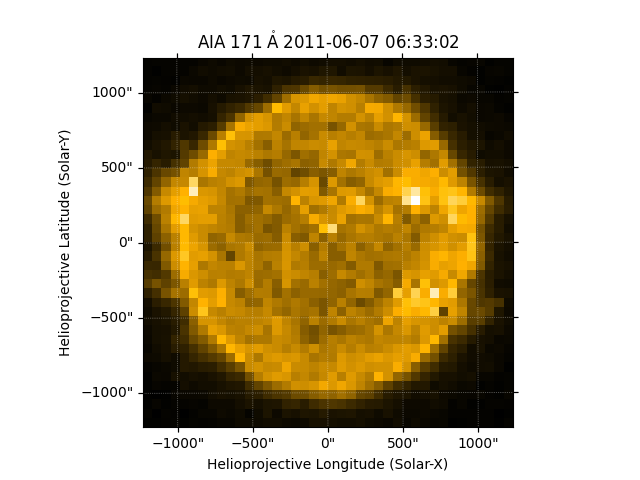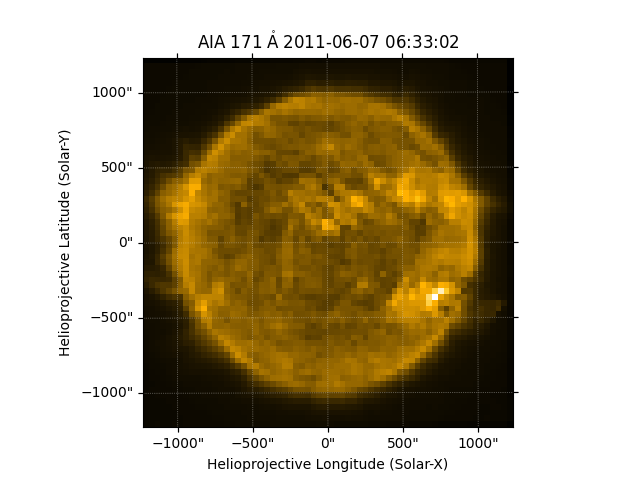# Resampling Maps#

How to resample a map using the resample method, which implements interpolation, or using superpixels, which combines pixels.

import matplotlib.pyplot as plt

import astropy.units as u

import sunpy.data.sample
import sunpy.map


aia_map = sunpy.map.Map(sunpy.data.sample.AIA_171_IMAGE)


To reduce the angular resolution of the map you can use the resample method, specifying the new dimensions in pixels. By default, this method uses linear interpolation but this can be changed with the method argument (‘nearest’, ‘linear’ or ‘spline’).

new_dimensions = [40, 40] * u.pixel
aia_resampled_map = aia_map.resample(new_dimensions)


Let’s plot the result.

fig = plt.figure()
aia_resampled_map.plot(axes=ax)
plt.show()Another way to resample is by using the superpixel method. This can be used to increase the signal to noise ratio by reducing the resolution of the image by combining pixels. This means that the new dimension must divide the original size exactly. For example you can reduce the AIA map resolution by a factor of 16.

new_dimensions = u.Quantity(aia_map.dimensions) / 16
aia_superpixel_map = aia_map.superpixel(new_dimensions)


Let’s plot the result.

fig = plt.figure()